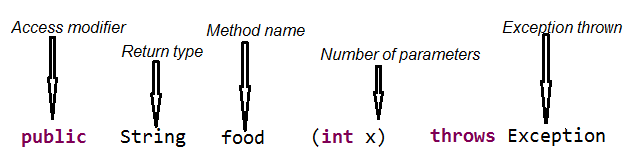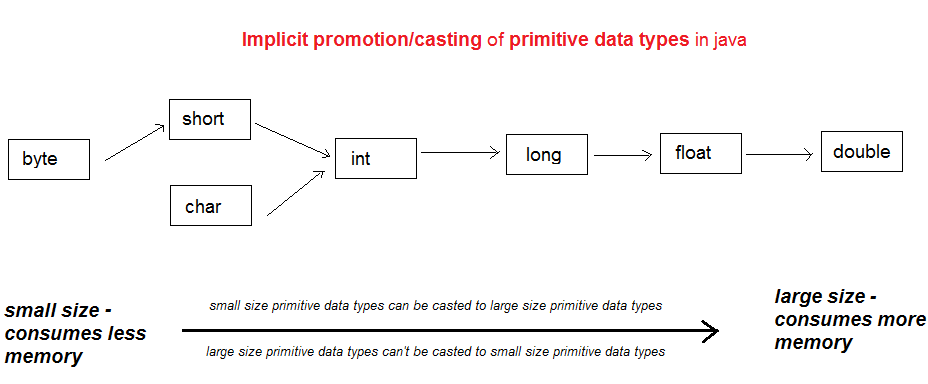Contents of page :
• Program 1 -  to overload methods by changing number of arguments.
• Program 2 - to overload methods by keeping same number of arguments but changing data data type of arguments
• Implicit casting/promotion of primitive Data type in java >
• Diagram of Implicit casting/promotion of primitive Data type in java >
• Tabular form Implicit casting/promotion of primitive Data type in java >
Now, let’s create programs to understand Implicit Data type casting/ promotion of primitive data types in java with method overloading in java >
• Program 5.1 -
• In the program, 2 is a int, 2 can be casted to double as well, but rather than casting compiler will call method with int as argument.
• Program 5.2 -
• In the program, 2 is a int, 2 can be casted to double and float as well, but rather than casting to double compiler will cast 2 to float and call method with float as argument.
• Program 5.3 -
• In the program, 2 is a int, 2 can be casted to double but cannot be casted to short, compiler will cast 2 to double and call method with double as argument.
• Data types are not demoted Implicitly, though they may be demoted explicitly, which may cause data loss.

• Example Program 6 - If we want to demote double to int, we will have to add explicit cast. In explicit cast we lost decimal value of 2.2 and are left with 2

When a class have same method name with different argument, than it is called method overloading.

Method overloading enables consistency in the naming of methods which logically perform almost similar tasks and the only difference is in number of arguments. Method overloading enables same method name to be reused in program.

Example -
 /*    * Method to calculate sum of 2 arguments    */    public void sum(int x, int y) {           System.out.println("sum of 2 arguments = "+ (x+y));    }    /*    * Method to calculate sum of 3 arguments    */    public void sum(int x, int y, int z) {           System.out.println("sum of 3 arguments = "+ (x+y+z));    }
sum() method logically perform almost similar tasks and the only difference is in number of arguments. Method overloading enables same method name sum() to be reused in program.

1. changing number of arguments, or  (In Program 1 below)
2. keeping same number of arguments but changing data data type of arguments.  (In Program 2 below)

Method definition is formed by using following 5 terms -1. Access modifier - Does not matter.

1. Return type - Does not matter.

1. Method name - same method name.

1. Number of parameters in java - different number of parameters

1. Exception thrown - Does not matter.

1. Call to overloaded method is bonded at compile time.

1. Method overloading concept is also known as compile time polymorphism in java.

1. Java does not allow overloading by changing the return type, though overloaded methods can change the return type.

1. Method overloading is generally done in same class but can also be done in SubClass (In Program 3 below)

1. private methods can be overloaded in java.

1. final methods can be overloaded in java.

1. Main method can also be overloaded in java (In Program 4 below)

1. Both Static and instance method can be overloaded in java.  (In Program 1 below, we have overloaded instance method, likewise we could also overload static method)

Program 1 -  to overload methods by changing number of arguments.
 /** Copyright (c), AnkitMittal JavaMadeSoEasy.com */ public class MyClass {    /*    * Method to calculate sum of 2 arguments    */    public void sum(int x, int y) {           System.out.println("sum of 2 arguments = "+ (x+y));    }    /*    * Method to calculate sum of 3 arguments    */    public void sum(int x, int y, int z) {           System.out.println("sum of 3 arguments = "+ (x+y+z));    }    public static void main(String[] args) {           MyClass obj = new MyClass();           obj.sum(2, 3); // will call method to calculate sum of 2 arguments           obj.sum(2, 4, 3); // will call method to calculate sum of 3 arguments    } } /*OUTPUT sum of 2 arguments = 5 sum of 3 arguments = 9 */

Program 2 - to overload methods by keeping same number of arguments but changing data data type of arguments
 /** Copyright (c), AnkitMittal JavaMadeSoEasy.com */ public class MyClass {    /*    * Method to calculate sum of 2 int type arguments    */    public void sum(int x, int y) {           System.out.println("sum of 2 int type arguments = "+ (x+y));    }    /*    * Method to calculate sum of 2 double type arguments    */    public void sum(double x, double y) {           System.out.println("sum of 2 double type arguments = "+ (x+y));    }    public static void main(String[] args) {           MyClass obj = new MyClass();           obj.sum(2, 3); // will call method to calculate sum of 2 int type arguments           obj.sum(1.2, 2.3); // will call method to calculate sum of 2 double type arguments    } } /*OUTPUT sum of 2 int type arguments = 5 sum of 2 double type arguments = 3.5 */

 class SuperClass{    public void sum(int x, int y) {           System.out.println("sum of 2 int type arguments = "+ (x+y));    } } class SubClass extends SuperClass{    public void sum(double x, double y) {           System.out.println("sum of 2 double type arguments = "+ (x+y));    } } /** Copyright (c), AnkitMittal JavaMadeSoEasy.com */ public class MyClass {    public static void main(String[] args) {           SubClass obj = new SubClass();           obj.sum(2, 1);           obj.sum(2.1, 3.4);    } } /*OUTPUT sum of 2 int type arguments = 3 sum of 2 double type arguments = 5.5 */
In the above program, method with 2 double type arguments of SubClass overloaded method with 2 double type arguments of SuperClass.

 /** Copyright (c), AnkitMittal JavaMadeSoEasy.com */ public class MyClass {    public static void main(String[] args) {           System.out.println("main(String[] args)");           main();    }    public static void main(){           System.out.println("main()");    } } /*OUTPUT main(String[] args) main() */
When JVM loads MyClass it finds out main method with signature = [public static void main(String[] args)].

Implicit casting/promotion of primitive Data type in java >
It’s very important from ocjp exam point of view.

Diagram of Implicit casting/promotion of primitive Data type in java >Tabular form Implicit casting/promotion of primitive Data type in java >
 Primitive Data type Can be implicitly casted/promoted to byte short, int, long, float, double short int, long, float, double char int, long, float, double int long, float, double long float, double float double

Now, let’s create programs to understand Implicit Data type casting/ promotion of primitive data types in java with method overloading in java >

Program 5.1 -
In the program, 2 is a int, 2 can be casted to double as well, but rather than casting compiler will call method with int as argument.
 /** Copyright (c), AnkitMittal JavaMadeSoEasy.com */ public class MyClass {    static void m(double x) {           System.out.println("double");    }    static void m(int x) {           System.out.println("int");    }       public static void main(String args[]) {           m(2);    } } /*OUTPUT int */

Program 5.2 -
In the program, 2 is a int, 2 can be casted to double and float as well, but rather than casting to double compiler will cast 2 to float and call method with float as argument.
 public class MyClass {    static void m(double x) {           System.out.println("double");    }    static void m(float x) {           System.out.println("float");    }       public static void main(String args[]) {           m(2);    } } /*OUTPUT float */

When an exact match is not found for parameter then compiler finds the method with the smallest argument that is wider than the parameter. This concept is also known as widening of data type.
Example - In the above program, an exact match is not found for parameter (int) then compiler finds the method with the smallest argument (float rather than double) that is wider than the parameter(int). int is widened to float.

Program 5.3 -
In the program, 2 is a int, 2 can be casted to double but cannot be casted to short, compiler will cast 2 to double and call method with double as argument.
 public class MyClass {    static void m(double x) {           System.out.println("double");    }    static void m(short x) {           System.out.println("short");    }       public static void main(String args[]) {           m(2);    } } /*OUTPUT double */

In the above program,  int is widened to double.

Program 5.3 -
In the program, 2 is a int, 2 can’t be casted to either short or byte, so we will face compilation error
 public class MyClass {    static void m(byte x) {           System.out.println("double");    }    static void m(short x) {           System.out.println("short");    }       public static void main(String args[]) {           m(2);  //compilation error    } } /*OUTPUT */

Data types are not demoted Implicitly, though they may be demoted explicitly, which may cause data loss.

Example Program 6 - If we want to demote double to int, we will have to add explicit cast. In explicit cast we lost decimal value of 2.2 and are left with 2
 public class MyClass {    public static void main(String args[]) {               double d=2.2;           int i=(int) d;           System.out.println(i);    } } /*OUTPUT 2 */